Next: Gary Walsh - Old Up: Number Theory / Théorie Previous: Gael Rémond - Theta

## D. Roy - Criteria of algebraic independence and approximation by hypersurfaces

 D. ROY, University of Ottawa, Ottawa, Ontario  K1N 6N5, Canada Criteria of algebraic independence and approximation by hypersurfaces

Given a point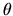in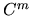, a fundamental problem is how close one can approximateby a point of an algebraic variety of dimension d, defined over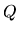, with degree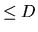and logarithmic height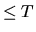. The problem has a different flavor whether, for a fixed d, one wants an estimate valid for a pair (D,T) or for infinitely many pairs (Dn,Tn) chosen from a given non-decreasing sequence of positive integers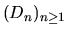, and a given non-decreasing unbounded sequence of positive real numbers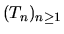with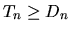for each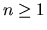. In a joint work with Michel Laurent, we analyze the second type of problem when d=m-1. We show that, for infinitely many indices n, there exists a nonzero polynomial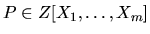of degree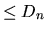whose coefficients have absolute value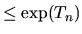, such that Padmits at least one zero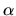inwith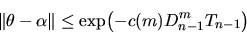for some positive constant c(m) which depends only on m. This follows from a new criteria of algebraic independence with multiplicities. The object of the talk is to explain the link between the two results.Next: Gary Walsh - Old Up: Number Theory / Théorie Previous: Gael Rémond - Theta